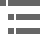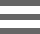## ResolutionThe resolution of an optical microscope is defined as the shortest distance between two points on a specimen that can still be distinguished by the observer or camera system as separate entities. An example of this important concept is presented in the figure below (Figure 1), where point sources of light from a specimen appear as Airy diffraction patterns at the microscope intermediate image plane.

The limit of resolution of a microscope objective refers to its ability to distinguish between two closely spaced Airy disks in the diffraction pattern (noted in the figure). Three-dimensional representations of the diffraction pattern near the intermediate image plane are known as the point spread function, and are illustrated in the lower portion of Figure 1. The specimen image is represented by a series of closely spaced point light sources that form Airy patterns and is illustrated in both two and three dimensions.

Resolution is a somewhat subjective value in optical microscopy because at high magnification, an image may appear unsharp but still be resolved to the maximum ability of the objective. Numerical aperture determines the resolving power of an objective, but the total resolution of the entire microscope optical train is also dependent upon the numerical aperture of the substage condenser. The higher the numerical aperture of the total system, the better the resolution.

Correct alignment of the microscope optical system is also of paramount importance to ensure maximum resolution. The substage condenser must be matched to the objective with respect to numerical aperture and adjustment of the aperture iris diaphragm for accurate light cone formation and specimen illumination. The wavelength spectrum of light used to image a specimen is also a determining factor in the degree of resolution afforded by the microscope. Shorter wavelengths are capable of resolving details to a greater degree than are the longer wavelengths. There are several equations that have been derived to express the relationship between numerical aperture, wavelength, and resolution:

Where r is resolution (the smallest resolvable distance between two objects), NA is a general term for the microscope numerical aperture, λ is the imaging wavelength, NA(obj) equals the objective numerical aperture, and NA(cond) is the condenser numerical aperture. Notice that equation (1) and (2) differ by the multiplication factor, which is 0.5 for equation (1) and 0.61 for equation (2). These equations are based upon a number of factors (including a variety of theoretical calculations made by optical physicists) to account for the behavior of objectives and condensers, and should not be considered an absolute value of any one general physical law. In some instances, such as confocal and fluorescence microscopy, the resolution may actually exceed the limits placed by any one of these three equations. Other factors, such as low specimen contrast and improper illumination may serve to lower resolution and, more often than not, the real-world maximum value of r (about 0.25 µm using a mid-spectrum wavelength of 550 nanometers) and a numerical aperture of 1.35 to 1.40 are not realized in practice. The following table (Table 1) provides a list resolution (r) and numerical aperture (NA) values by objective magnification and correction.

When the microscope is in perfect alignment and has the objectives appropriately matched with the substage condenser, then we can substitute the numerical aperture of the objective into equations (1) and (2), with the added result that equation (3) reduces to equation (2). An important fact to note is that magnification does not appear as a factor in any of these equations, because only numerical aperture and wavelength of the illuminating light determine specimen resolution.

As we have mentioned (and can be seen in the equations) the wavelength of light is an important factor in the resolution of a microscope. Shorter wavelengths yield higher resolution (lower values for r) and visa versa. The greatest resolving power in optical microscopy is realized with near-ultraviolet light, the shortest effective imaging wavelength. Near-ultraviolet light is followed by blue, then green, and finally red light in the ability to resolve specimen detail. Under most circumstances, microscopists use white light generated by a tungsten-halogen bulb to illuminate the specimen. The visible light spectrum is centered at about 550 nanometers, the dominant wavelength for green light (our eyes are most sensitive to green light). It is this wavelength that was used to calculate resolution values in the Table 1. The numerical aperture value is also important in these equations and higher numerical apertures will also produce higher resolution. The effect of the wavelength of light on resolution, at a fixed numerical aperture (0.95), is listed in Table 2, with longer wavelengths producing lowered degrees of resolution.

The resolving power of a microscope is the most important feature of the optical system and influences the ability to distinguish between fine details of a particular specimen. As discussed above, the primary factor in determining resolution is the objective numerical aperture, but resolution is also dependent upon the type of specimen, coherence of illumination, degree of aberration correction, and other factors such as contrast enhancing methodology either in the optical system of the microscope or in the specimen itself. In the final analysis, resolution is directly related to the useful magnification of the microscope and the perception limit of specimen detail.

### Contributing Author

Michael W. Davidson - National High Magnetic Field Laboratory, 1800 East Paul Dirac Dr., The Florida State University, Tallahassee, Florida, 32310.

# Microscopy U - The source for microscopy education•••